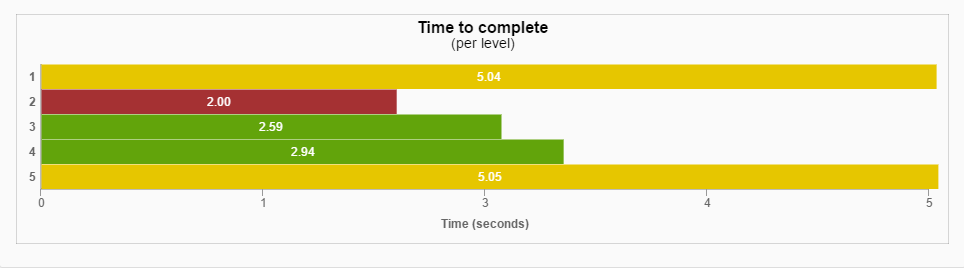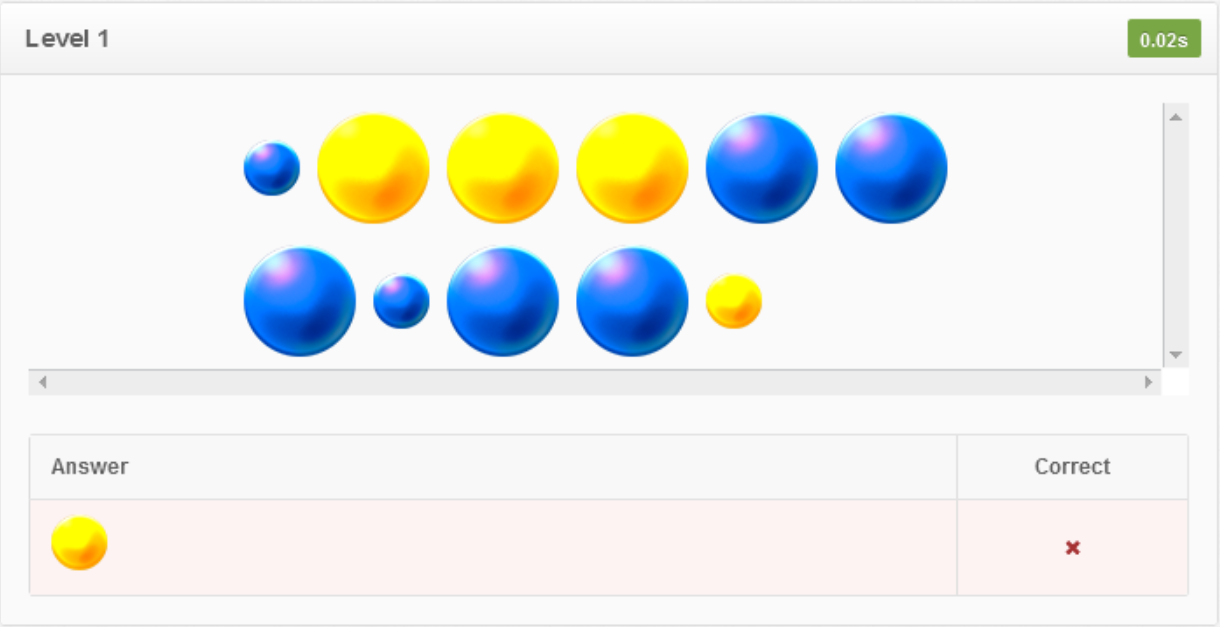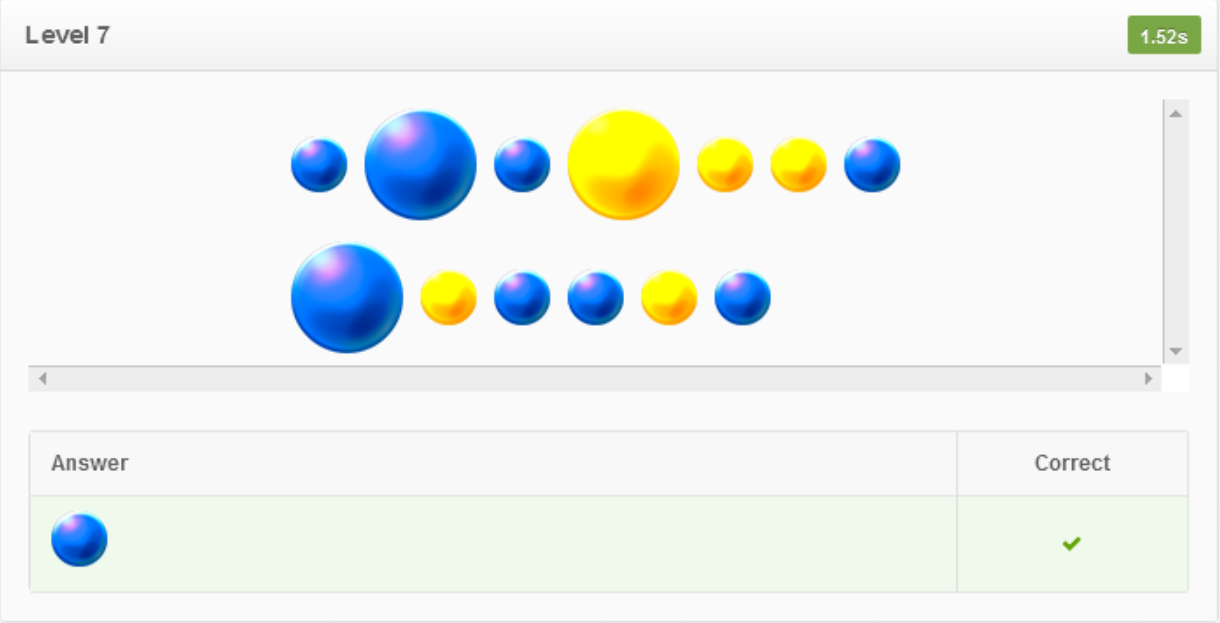### Bilisius

The “Bilisius” game fosters student’s ability to quickly identify the larger set of colored marbles without counting. The student becomes a magician avatar who sidewalks and tries to solve problems. The student views a cluster of two sets of yellow and blue marbles at the top of the screen. They need to quickly decide (without having enough time to count) which set has more marbles than the other. They respond by moving their body left or right to the matching colored blue or yellow small carpet. The teacher/therapist can choose whether the marbles of different colors will have the same size or not as well as total number of marbles that will be shown to the student. The more marbles (up to 20) that appear, the more difficult the game becomes. Also, the teacher/therapist can make the game more challenging by adding game lives and an active timer. This game strengthens the children’s visual perception, promotes the ability to make rapid, accurate, and confident judgments. This game improves the concept of more and less using visual judgement. Ready for becoming a magician and play with the colored marbles?

## Supported goals in Common CoreCaliforniaFloridaNevadaNew YorkNorth CarolinaPennsylvaniaSouth CarolinaTenesseeTexasVirginia

CCSS.MATH.CONTENT.K.MD.B.3
Math
Classify objects into given categories; count the numbers of objects in each category and sort the categories by count.
CCSS.MATH.CONTENT.PK.CC.5
Math
Identify whether the number of objects in one group is more, less, greater than, fewer, and/or equal to the number of objects in another group, e.g., by using matching and counting strategies (up to 5 objects).
CA.MATH.K.MD.3
Math
Classify objects and count the number of objects in each category. Classify objects into given categories; count the numbers of objects in each category and sort the categories by count. (Limit category counts to be less than or equal to 10.)
OEL.FL.MATH.PK.CC.5
Math
Identify whether the number of objects in one group is more, less, greater than, fewer, and/or equal to the number of objects in another group, e.g., by using matching and counting strategies (up to 5 objects).
MAFS.K.MD.2.AP.3a
Math
Sort objects by characteristics (e.g., big/little, colors, shapes).
NVAC.MATH.K.MD.B.3
Math
Classify objects and count the number of objects in each category. Classify objects into given categories
NY.MATH.K.MD.3
Math
Classify objects into given categories; count the numbers of objects in each category and sort the categories by count
NY.MATH.PK.CC.5
Math
Identify whether the number of objects in one group is more, less, greater than, fewer, and/or equal to the number of objects in another group, e.g., by using matching and counting strategies (up to 5 objects).
NC.MATH.K.MD.3
Math
Classify objects into given categories; count the numbers of objects in each category and sort the categories by count.
PA.CC.MATH.PK.CC.5
Math
Identify whether the number of objects in one group is more, less, greater than, fewer, and/or equal to the number of objects in another group, e.g., by using matching and counting strategies (up to 5 objects).
PA.CC.MATH.K.MD.B.3
Math
Classify objects into given categories; count the numbers of objects in each category and sort the categories by count.
SC.MATH.PK.CC.5
Math
Identify whether the number of objects in one group is more, less, greater than, fewer, and/or equal to the number of objects in another group, e.g., by using matching and counting strategies (up to 5 objects).
SC.MATH.K.MDA.3
Math
Sort and classify data into 2 or 3 categories with data not to exceed 20 items in each category.
No supported goals.
TEKS.MATH.PK.CC.5
Math
Identify whether the number of objects in one group is more, less, greater than, fewer, and/or equal to the number of objects in another group, e.g., by using matching and counting strategies (up to 5 objects).
No supported goals.

## Settings

### I CAN statement

Music

Disable/enable background music

• On
• Off
Sound effects

Disable/enable sound effects while selecting an item.

• On
• Off

I CAN understand that there are sounds when I choose an item.

Audio message
• On
• Off
Select hand
• Left
• Right

I CAN select the “Play Button” with my left hand.

Time to select item

Time to remain stable to select an item for initiating the game

Reduce the time if the child shows arm stability

• 0.5 sec
• 1 sec
• 1.5 sec
• 2 secs
• 2.5 secs
• 3 secs

I CAN hold my left hand still for 3 seconds to select an item.

Timer

Time to finish selecting the side

Disable this if the student feels pressure

The green lights on the tree are the number of seconds left

• On
• Off
Timer value
• 3 secs
• 4 secs
• 5 secs

I CAN answer 1 question in five seconds.

Content language
• English
• Greek
• Spanish
Number of questions

These show the number of questions for each game.

• 5 questions
• 10 questions
• 15 questions
• 20 questions

I CAN answer 5 questions correctly.

Marble size

Determine whether the marble size should be the same or mixed size.

• Same
• Different

I CAN give correct answers even though the marble sizes are different.

Amount of marbles

This function allows teachers to choose the theme that the student will work on.

• Few
• Some
• Many

I CAN determine a fewer amount of marbles.

game_lifes

Enable or disable game lives Game lives are filters on the right of the screen.

• On
• Off

I CAN notice that the filters went off when I am wrong. I CAN play the game with game lives.

Game difficulty

Determine the ratio in the amount of yellow and blue marbles

• Easy
• Medium
• Hard

I CAN win a game in an easy level.

## ReportsPie chart data

·      This shows statistics of how many times the student gave wrong answers or no answer.

The Time is shown on the top right corner. The teacher can use the time to tell whether the task is challenging enough for the students.Graph data

·      This shows the amount of time the student spent on each question, which question was wrong or unanswered. In this case, the student spent the longest time on question 4 (2, 94 s).

The data can help the teacher to see whether there is a certain pattern that the student face difficulty in finding the right answer.The student got the question no.1 wrong in 0.02s. This might be an error due to unfamiliarity with the game.

The result shows what the question looks like, the correct answer, and the student’s answer.The student got the question no.7 right in 1.52s which is similar in term of timing with questions no. 5 and 8. This might indicate that the student understands the game and the mathematical concept.